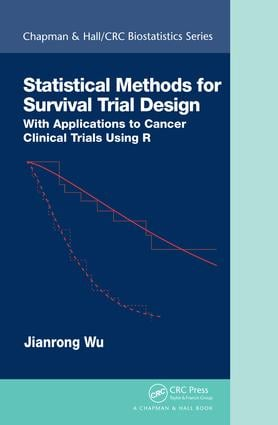# Statistical Methods for Survival Trial Design

## With Applications to Cancer Clinical Trials Using R, 1st Edition

Chapman and Hall/CRC

257 pages

##### Purchasing Options:\$ = USD
Hardback: 9781138033221
pub: 2018-06-18
\$119.95
x
eBook (VitalSource) : 9780429470172
pub: 2018-06-14
from \$28.98

FREE Standard Shipping!

### Description

Statistical Methods for Survival Trial Design: With Applications to Cancer Clinical Trials Using R provides a thorough presentation of the principles of designing and monitoring cancer clinical trials in which time-to-event is the primary endpoint.Traditional cancer trial designs with time-to-event endpoints are often limited to the exponential model or proportional hazards model. In practice, however, those model assumptions may not be satisfied for long-term survival trials.

This book is the first to cover comprehensively the many newly developed methodologies for survival trial design, including trial design under the Weibull survival models; extensions of the sample size calculations under the proportional hazard models; and trial design under mixture cure models, complex survival models, Cox regression models, and competing-risk models. A general sequential procedure based on the sequential conditional probability ratio test is also implemented for survival trial monitoring. All methodologies are presented with sufficient detail for interested researchers or graduate students.

### Reviews

". . . this book provides a comprehensive introduction to statistical methods in cancer of sample size calculations and survival clinical trial designs from the classical techniques to the newly proposed formulae such as the mixture cure model and a group sequential trial design. This book has a vast list of citations and is an excellent reference for statisticians performing oncology research in the pharmaceutical industry or in other settings, and for graduate students in biostatistics or in related fields." ~ Journal of Biopharmaceutical Statistics

Preface

List of Figures

List of Tables

1. Introduction to Cancer Clinical Trials

General Aspects of Cancer Clinical Trial Design

Study Objectives

Treatment Plan

Eligibility Criteria

Statistical Considerations

Statistical Aspects of Cancer Survival Trial Design

Randomization

Stratification

Blinding

Sample Size Calculation

2. Survival Analysis

Survival Distribution

Exponential Distribution

Weibull Distribution

Gamma Distribution

Gompertz Distribution

Log-Normal Distribution

Log-Logistic Distribution

Survival Data

Fitting the Parametric Survival Distribution

Kaplan-Meier Estimates

Median Survival Time

Log-Rank Test

Cox Regression Model

3. Counting Process and Martingale_

Basic Convergence Concepts

Counting Process Definition

Martingale Central Limit Theorem

Counting Process Formulation of Censored Survival Data

4. Survival Trial Design Under the Parametric Model

Introduction

Weibull Model

Test Statistic

Distribution of the MLE test

Sample Size Formula

Sample Size Calculation

Accrual Duration Calculation

Example and R code

5. Survival Trial Design Under the Proportional Hazards Model

Introduction

Proportional Hazards Model

Asymptotic Distribution of the Log-rank Test

Schoenfeld Formula

Rubinstein Formula

Freedman Formula

Comparison

Sample Size Calculation Under Various Models

Example

Optimal Properties of the Log-Rank Test_

Optimal Sample Size Allocation

Optimal Power

Precise Formula

Exact Formula

6. Survival Trial Design Under the Cox Regression Model

Introduction

Test Statistics

Asymptotic Distribution of the Score Test_

Sample Size Formula

7. Complex Survival Trial Design

Extension of the Freedman Formula

Example and R code

Lakatos Formula

Markov Chain Model with Simultaneous Entry

Computation Formulae

Markov Chain Model with Staggered Entry

Examples and R code

8. Survival Trial Design Under the Mixture Cure Model

Introduction

Testing Differences in Cure Rates

Mixture Cure Model

Asymptotic Distribution

Sample Size Formula

Optimal Log-Rank Test

Comparison

Example and R code

Conclusion

Testing Differences in Short- and Long-Term Survival

Hypothesis Testing

Ewell and Ibrahim Formula

Simulation

Example and R code

Conclusion

9. A General Group Sequential Procedure

Brownian Motion

Sequential Conditional Probability Ratio Test

Operating Characteristics

Probability of Discordance

SCPRT Design

10. Sequential Survival Trial Design

Introduction

Sequential Procedure for the Parametric Model

Sequential Wald Test

SCPRT for the Parametric Model

Sequential Procedure for the Proportional Hazard Model

Sequential Log-Rank Test

Information Time

SCPRT for the PH Model

11. Sequential Survival Trial Design Using Historical Controls

Introduction

Sequential Log-Rank Test with Historical Controls

Sample Size Calculation

Information Time

Group Sequential Procedure

Conclusion

12. Some Practical Issues in Survival Trial Design

Parametric vs Nonparametric Model

Nonproportional Hazards Model

Accrual Patterns

Mixed Populations

Loss to Follow-Up

Noncompliance and Drop-In

Competing Risk

A Likelihood Function For the Censored Data

B Probability of Failure Under Uniform Accrual

C Verification of the Minimum Sample Size Conditions

D R Codes for the Sample Size Calculations

E Derivation of the Asymptotic Distribution

F Derivation of Equations for Chapter

Bibliography

Index

### About the Author

Jianrong (John) Wu is a professor in the Division of Cancer Biostatistics, Department of Biostatistics, Markey Cancer Center, University of Kentucky. He has more than 15 years’ experience of designing and conducting cancer clinical trials at St. Jude Children’s Research Hospital and has developed several novel statistical methods for designing phase II and phase III survival trials.

### Subject Categories

##### BISAC Subject Codes/Headings:
MAT029000
MATHEMATICS / Probability & Statistics / General
MED071000
MEDICAL / Pharmacology
MED107000
MEDICAL / Genetics